• 2018-04-25 15:10:17
%from this on

fun=@(p,x) p(1)./x.*exp(-((log(x)-p(2))/p(3)).^2/2);%对数正态分布密度函数

x=(0:0.02:6)*1e4;

y=fun([3e5,8.3,0.6],x)+rand(size(x)).*exp(-(x/2e4).^2)*20;

%原来你的图可能是用hist(data,n)画出来的

%就是将data数据分n个区间统计，画柱状图

%这里要保留hist的数据

%原来的语句要返回x y值

%[y x]=hist(data,n);

%这里因为没有数据所以用上边两句模拟x，y数据

bar(x,y,1);hold on;%根据x，y数据画柱状图

[maxy ind]=max(y);

p=nlinfit(x,y,fun,[maxy*x(ind),log(x(ind)),1]);%拟合

%p(1)~和幅度有关    p(2)~mu    p(3)~sigma

yfit=fun(p,x); %计算拟合曲线

plot(x,yfit,'r','linewidth',2);

xmax=exp(p(2)-p(3)^2);%计算分布极大分布处和值 x=exp(mu-sigma^2);

ymax=fun(p,xmax);

plot([xmax xmax],[0 ymax],'g','linewidth',2);

xmean=exp(p(2)+p(3)^2/2);%计算期望值 x=exp(mu+sigma^2/2)

ymean=fun(p,xmean);

plot([xmean xmean],[0 ymean],'c','linewidth',2);

hold off;

xlim([min(x) max(x)]);

xlabel('BC浓度（ng/m^3）');

ylabel('频数');

legend('统计数据',['对数正态分布:\mu=' num2str(p(2)) ',\sigma=' num2str(p(3))],...

['极大概然分布位置:x=' num2str(xmax)],['期望值位置:x=' num2str(xmean)]);

text(xmean+10000,ymean+10,'$y=\frac{A}{x}e^{-\frac{(lnx-\mu)^2}{2\sigma^2}}$',...
'interpreter','latex','FontSize',18);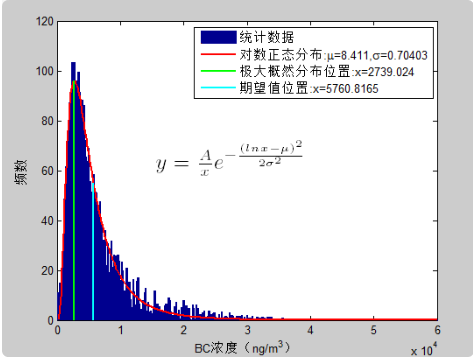参考：

no1:https://zhidao.baidu.com/question/753137168512272564.html

no2:https://zhidao.baidu.com/question/327808626938436325.html?qbl=relate_question_1&word=matlab%20%D2%BB%D7%E9%CA%FD%BE%DD%C4%E2%BA%CF%D5%FD%CC%AC%B7%D6%B2%BC%C7%FA%CF%DF%B5%C4%B3%CC%D0%F2

no3:https://zhidao.baidu.com/question/327808626938436325.html?qbl=relate_question_1&word=matlab%20%D2%BB%D7%E9%CA%FD%BE%DD%C4%E2%BA%CF%D5%FD%CC%AC%B7%D6%B2%BC%C7%FA%CF%DF%B5%C4%B3%CC%D0%F2

更多相关内容
• 包含对正态分布和对数正态分布的直方图数据的拟合。 虽然仍然需要拟合工具箱，但参数的调整方式使 Matlab 可以实际拟合值（例如非常小的 x 值）！ 函数返回 mu 和 sigma 参数、缩放因子（对于非单位分布）和拟合...
• 06-jun-05 将三种概率分布（正态、对数正态、威布尔）之一拟合到输入数据向量。 如果将分布指定为“最佳”，则会自动选择最适合数据的分布。 输入如果 nargin==1，则提示“分布”并交互输入 数据 - nx 1 或 1 xn ...
• 我想使用Scipy拟合对数正态分布。...拟合对数正态分布使用Scipy与Matlab下面是Matlab代码我用适合我的数据：% Read input data (one value per line)x = [];fid = fopen(file_path, 'r'); % reading ...

我想使用Scipy拟合对数正态分布。我之前已经使用Matlab来完成它，但由于需要将应用程序扩展到统计分析之外，我正在试图在Scipy中重现拟合值。拟合对数正态分布使用Scipy与Matlab

下面是Matlab代码我用适合我的数据：

% Read input data (one value per line)

x = [];

fid = fopen(file_path, 'r'); % reading is default action for fopen

if fid == -1

disp('[ERROR] Unable to open data file.')

else

while ~feof(fid)

[x] = [x fscanf(fid, '%f', )];

end

c = fclose(fid);

if c == 0

disp('File closed successfully.');

else

disp('[ERROR] There was a problem with closing the file.');

end

end

[f,xx] = ecdf(x);

y = 1-f;

parmhat = lognfit(x); % MLE estimate

mu = parmhat(1);

sigma = parmhat(2);

而这里的拟合图：现在，这里是我的Python代码实现同样的目的：

import math

from scipy import stats

from statsmodels.distributions.empirical_distribution import ECDF

# The same input is read as a list in Python

ecdf_func = ECDF(degrees)

x = ecdf_func.x

ccdf = 1-ecdf_func.y

# Fit data

shape, loc, scale = stats.lognorm.fit(degrees, floc=0)

# Parameters

sigma = shape # standard deviation

mu = math.log(scale) # meanlog of the distribution

fit_ccdf = stats.lognorm.sf(x, [sigma], floc=1, scale=scale)

下面是使用Python代码的配合。正如你看到的，代码两套能够产生良好的配合，至少在视觉上来讲的。

问题是估计参数mu和sigma存在巨大差异。

来自Matlab：mu = 1.62 sigma = 1.29。 Python：mu = 2.78 sigma = 1.74。

为什么会有这样的差异？

注意：我已经仔细检查过两套数据都是正确的,一样。分数相同，分布相同。

非常感谢您的帮助！提前致谢。

其他信息：

import scipy

import numpy

import statsmodels

scipy.__version__

'0.9.0'

numpy.__version__

'1.6.1'

statsmodels.__version__

'0.5.0.dev-1bbd4ca'

Matlab的版本是R2011b。

版：

正如下面的回答证明，故障在于SciPy的0.9。我能够使用Scipy 11.0从Matlab重现mu和sigma结果。

一个简单的方法来更新您的SciPy的是：

如果你不具备PIP(你应该！)：

sudo apt-get install pip

2013-03-26

Mike

+1

看看这两组数据点，它们看起来相当不同(例如，比较右下角蓝色圆圈的位置)。如果数据不相同，则没有理由认为适合。 –

2013-03-26 06:29:18

+0

两组数据*完全相同。我已经彻底检查过，以确保事实并非如此。这些图显示略有不同，因为我用来在Matlab中绘制的代码是非库代码。无论如何，要点是所拟合的数据完全相同，因此它们应该产生相同的平均值和标准偏差值。 –

2013-03-26 06:40:59

+0

我很抱歉，但我不买这个(除非情节是关闭的)。只是直观地比较两个图上最右边的点的横坐标，看看它们是*非常*不同。如果您肯定数据是相同的，请将它与您用于将其读入Python和MATLAB的代码一起包含它。 –

2013-03-26 06:44:34

展开全文• 本代码主要利用MATLAB工具实现MATLAB——绘制正态拟合曲线直方图，简单明了，易于理解
• 假设现有一个名为data的1x500的数据，这里我们使用normrnd随机生成一个正态分布的数据 data=normrnd(0,5,[1,500...方法一是直接使用histfit函数,histfit函数会直接生成一个带分布直方图的正态分布拟合 histfit(data

假设现有一个名为data的1x500的数据，这里我们使用normrnd随机生成一个正态分布的数据

data=normrnd(0,5,[1,500]);

可以使用histogram函数对齐分布进行绘制，这里我们把它分成30个bar，可以粗略看出接近正态分布

histogram(data,30);
xlim([-16,16]);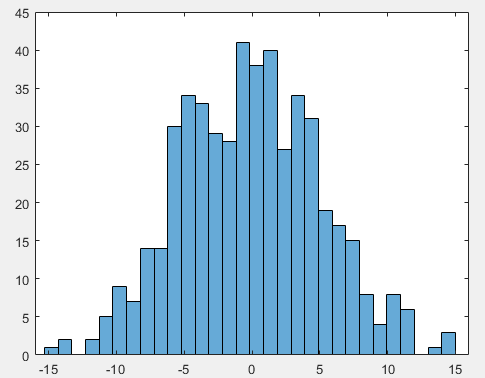如果想画的是频率图而不是频数图可以使用下面的代码

histogram(data,30,'Normalization','probability');

对其进行正态分布拟合，有两种方法可以选用

方法一是直接使用histfit函数,histfit函数会直接生成一个带分布直方图的正态分布拟合

histfit(data,30);
xlim([-16,16]);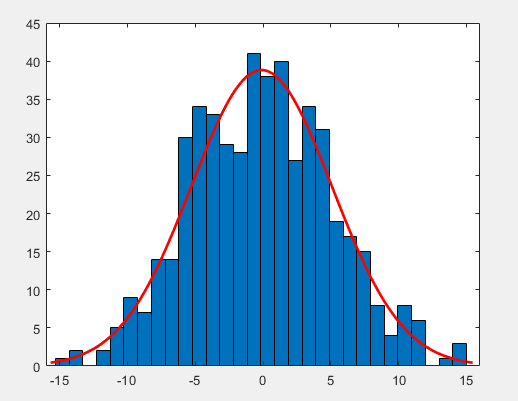方法二则是根据数据的均值和标准差直接拟合,使用函数normpdf生成一个对应的正态分布概率密度函数，乘以数据个数并把它画在上面的图里面，可以看到基本是一致的。这种方法可以单独画曲线，相对于方法一比较灵活。

hold on
x=-16:0.001:16;
fx=normpdf(x,mean(data),std(data));
plot(x,fx*500,'g-');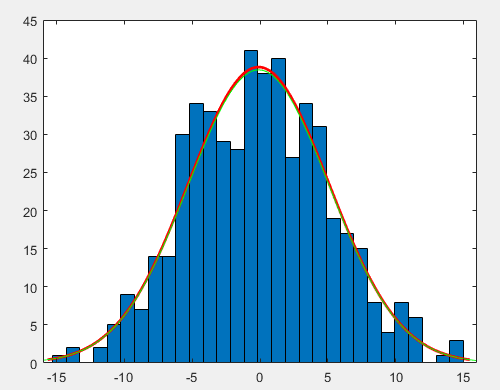展开全文统计模型
• I am trying to fit a lognormal distribution using ... I've already done it using Matlab before but because of the need to extend the application beyond statistical analysis, I am in the process of ...I am trying to fit a lognormal distribution using Scipy. I've already done it using Matlab before but because of the need to extend the application beyond statistical analysis, I am in the process of trying to reproduce the fitted values in Scipy.

Below is the Matlab code I used to fit my data:

% Read input data (one value per line)

x = [];

fid = fopen(file_path, 'r'); % reading is default action for fopen

if fid == -1

disp('[ERROR] Unable to open data file.')

else

while ~feof(fid)

[x] = [x fscanf(fid, '%f', )];

end

c = fclose(fid);

if c == 0

disp('File closed successfully.');

else

disp('[ERROR] There was a problem with closing the file.');

end

end

[f,xx] = ecdf(x);

y = 1-f;

parmhat = lognfit(x); % MLE estimate

mu = parmhat(1);

sigma = parmhat(2);

And here's the fitted plot: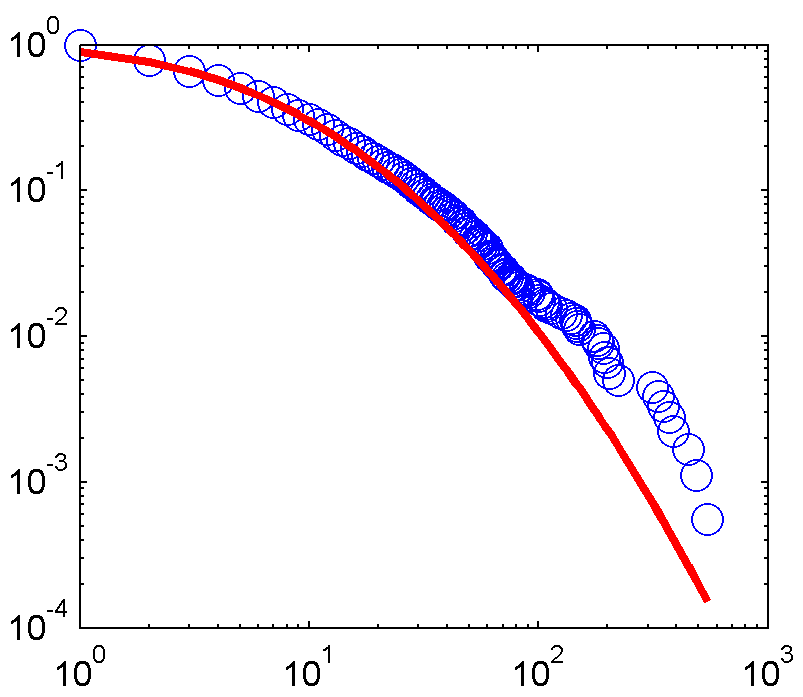Now here's my Python code with the aim of achieving the same:

import math

from scipy import stats

from statsmodels.distributions.empirical_distribution import ECDF

# The same input is read as a list in Python

ecdf_func = ECDF(degrees)

x = ecdf_func.x

ccdf = 1-ecdf_func.y

# Fit data

shape, loc, scale = stats.lognorm.fit(degrees, floc=0)

# Parameters

sigma = shape # standard deviation

mu = math.log(scale) # meanlog of the distribution

fit_ccdf = stats.lognorm.sf(x, [sigma], floc=1, scale=scale)

Here's the fit using the Python code.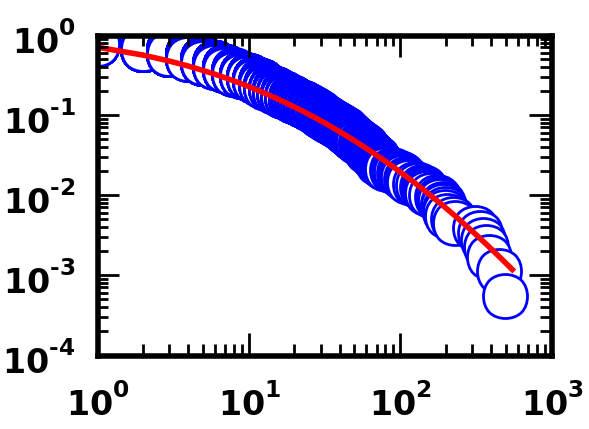As you can see, both sets of code are capable of producing good fits, at least visually speaking.

Problem is that there is a huge difference in the estimated parameters mu and sigma.

From Matlab: mu = 1.62 sigma = 1.29.

From Python: mu = 2.78 sigma = 1.74.

Why is there such a difference?

Note: I have double checked that both sets of data fitted are exactly the same. Same number of points, same distribution.

Other info:

import scipy

import numpy

import statsmodels

scipy.__version__

'0.9.0'

numpy.__version__

'1.6.1'

statsmodels.__version__

'0.5.0.dev-1bbd4ca'

Version of Matlab is R2011b.

Edition:

As demonstrated in the answer below, the fault lies with Scipy 0.9. I am able to reproduce the mu and sigma results from Matlab using Scipy 11.0.

An easy way to update your Scipy is:

If you don't have pip (you should!):

sudo apt-get install pip

解决方案

There is a bug in the fit method in scipy 0.9.0 that has been fixed in later versions of scipy.

The output of the script below should be:

Explicit formula: mu = 4.99203450, sig = 0.81691086

Fit log(x) to norm: mu = 4.99203450, sig = 0.81691086

Fit x to lognorm: mu = 4.99203468, sig = 0.81691081

but with scipy 0.9.0, it is

Explicit formula: mu = 4.99203450, sig = 0.81691086

Fit log(x) to norm: mu = 4.99203450, sig = 0.81691086

Fit x to lognorm: mu = 4.23197270, sig = 1.11581240

The following test script shows three ways to get the same results:

import numpy as np

from scipy import stats

def lognfit(x, ddof=0):

x = np.asarray(x)

logx = np.log(x)

mu = logx.mean()

sig = logx.std(ddof=ddof)

return mu, sig

# A simple data set for easy reproducibility

x = np.array([50., 50, 100, 200, 200, 300, 500])

# Explicit formula

my_mu, my_sig = lognfit(x)

# Fit a normal distribution to log(x)

norm_mu, norm_sig = stats.norm.fit(np.log(x))

# Fit the lognormal distribution

lognorm_sig, _, lognorm_expmu = stats.lognorm.fit(x, floc=0)

print "Explicit formula: mu = %10.8f, sig = %10.8f" % (my_mu, my_sig)

print "Fit log(x) to norm: mu = %10.8f, sig = %10.8f" % (norm_mu, norm_sig)

print "Fit x to lognorm: mu = %10.8f, sig = %10.8f" % (np.log(lognorm_expmu), lognorm_sig)

With the option ddof=1 in the std. dev. calculation to use the unbiased variance estimation:

In : x

Out: array([ 50., 50., 100., 200., 200., 300., 500.])

In : lognfit(x, ddof=1)

Out: (4.9920345004312647, 0.88236457185021866)

There is a note in matlab's lognfit documentation that says when censoring is not used, lognfit computes sigma using the square root of the unbiased estimator of the variance. This corresponds to using ddof=1 in the above code.

展开全文• 对数正态分布参数 mu 和 sigma，与数据本身均值m和方差v之间存在如下关系： 利用如下MATLAB代码，对上述关系进行了验证。 clc clear close all % 利用对数正态分布参数mu, sigma生成随机数 mu=1 sigma=0.3 a...均值算法
• 已经开发了以下代码和过程，以提供一个简单且完整的工具，用于从xls表格数据开始，根据对数正态分布和幂律分布自动实现对象拟合频率数据的自动化。 该程序使用外部资源对幂律分布进行拟合代码，我们将给出参考。 ...
• 02我们打开matlab之后，点击菜单栏里的“importdata”,准备加载我们需要统计分析的数据。03打开加载界面之后，我们找到我们要加载的数据文件，点击打开。04在加载的界面，我们将类型选择“matrix”矩阵列表，选择...
• Matlab正态分布检验：进行参数估计和假设检验时，一般老是假定整体服从正态分布，虽然在许多状况下这个假定是合理的，可是当要以此为前提进行重要的参数估计或假设检验，或者人们对它有较大怀疑的时候，就确有必要对...
• ## 对数正态分布(Log-Normal Distribution)

万次阅读 多人点赞 2017-11-01 16:02:07
2017.11.1 人要有发耻心和羞耻心，突然想到了这么一句话，MARK一个博客困惑了...- 相关分布快捷键 加粗 Ctrl + B 斜体 Ctrl + I 引用 Ctrl + Q 插入链接 Ctrl + L 插入代码 Ctrl + K 插入图片 Ctrl + G 提升标题 Ctrl
• 在用蒙特卡洛方法算不确定性，卡壳了。。思路是这样：1....那么问题来了：问题1 我知道怎么判断正态分布、指数分布等，求一个函数能求出一组数据的对数分布函数值。比如判断正态分布那个程序是：a...
• ## matlab拟合正态分布

千次阅读 2015-12-10 14:17:10
• 但是有时候得四个分布一起用，找来找去难免会有些麻烦，找到一篇文献：邓泽怀的硕士学位论文《基于实测数据的海杂波统计建模》，里面详细地讲解了上面提到的四种分布参数的矩估计、极大似然估计，并作了拟合优度检验...
• 函数 normfit() 格式 [muhat,sigmahat,muci,sigmaci] = normfit(X) ； [muhat,sigmahat,muci,... 函数格式：mle(观察值, ‘distribution’, ‘分布名称’) [paramhat,paramint]=mle(testdata,'distribution','norm')
• 时间：2017-03-16 20:30:20声明：欢迎被转载，记得注明出处~前言本文为曲线与分布拟合的一部分，主要介绍正态分布、拉普拉斯分布等常用分布拟合的理论推导以及代码实现。一、理论推导假设数据独立同分布。对于任意...
• 此函数根据文章中讨论的五个分布之一（幂定律，指数，对数正态，拉伸指数和具有截止的幂定律）生成随机分布的连续值。 使用信息包含在文件中； 在Matlab提示符下键入“ help randht”以获取更多信息。 plfit.m拟合幂...
• Jones 博士Johnson (1949) 开发了一种灵活的分布系统，它基于三个变换族，将观察到的非正态变量转换为符合标准正态分布的变量。 指数、逻辑和双曲正弦变换分别用于生成对数正态 (SL)、无界 (SU) 和有界 (SB) 分布。...
• 神说，要有正态分布，就有了正态分布。 神看正态分布是好的，就让随机误差服从了正态分布。 — 创世纪—数理统计 最小二乘法由天文学的问题产生，由法国的数学家勒让德Legendre)创造，但也有人说是高斯(Gauss)...
• 仿真输入之间的相关性蒙特卡罗模拟的设计决策之一是为随机输入选择概率分布。为每个变量选择一种分布往往很简单，但确定输入之间应该存在什么样的相关性却可能不那么简单。理想情况下，仿真的输入数据应反映要建模的...
• 在本文关于如何在R中进行贝叶斯分析。我们介绍贝叶斯分析，这个例子是关于职业足球比赛...在这种情况下，我们想知道θ的后验分布是什么样子的，这个分布的平均值是什么。为了做到这一点，我们将在三种情况下分析： ...R语言 贝叶斯 Poisson
• 对数正态 (lognfitc) 3. 物流（logistfitc） 4.对数物流（loglogistfitc） 5.极值（evfitc） 6.威布尔（wblfitc） 7.指数（expfitc） 8.伽玛（gamfitc） 9.瑞利（raylfitc） 输入参数： x：数据的两列矩阵。 例如...
• 累积分布函数cdf (CumulativeDistribution Function)背景知识：http://www.lifelaf.com/blog/?p=746语法y =cdf('name',x,A,B)y =cdf('name',x,A,B,C)y =cdf(pd,x)y =cdf(___,'upper')描述y =cdf('name',x,A) 计算...
• 用于超临界流体的混合物模型应用的其他代码（对数正态-中型混合物，概率分类）将很快上载。 如果您使用这些代码进行发布，请引用以下文章之一。  Yoon，TJ，Ha，MY，Lee，WB，＆Lee，Y.-W.。 （2017），超临界...
• 作者：桂。 时间：2017-03-11 06:45:46 ... 声明：欢迎转载，不过记得注明...数据拟合中，最常用的两个就是曲线拟合（curve fitting）与分布拟合（distribution fitting），不求面面俱到，只希望串出一个思路，篇目如...
• 尝试的分布是指数分布、正态分布、三角分布、均匀分布、伽玛分布、对数正态分布、泊松分布、威布尔分布。 用户可以输入第二个参数来选择高级特征来处理非数值输入向量/矩阵。 对每个拟合的分布进行 KS 检验。 选择 ...
• 话题：matlab拟合函数怎么搞？？多谢大神相助回答：x=[-20,-15,-10,-5,0,5,10,15,20,25,30,35,40,45,50,55,60,65,0,5,0,5,0,5,100,105,110,115,120]; y=[43.463,363.624,25.01,211.1656,163.006,126.45,.45...
• ## MATLAB各种概率分布画图(转载)

万次阅读 多人点赞 2013-12-13 22:40:12
如果数据来自正态分布，则图形显示为直线，而其它分布可能在图中产生弯曲。 例 4-53 >>X=normrnd(0,1,50,1); >> normplot(X)   图 4-12 4.6.5 绘制威布尔(Weibull)概率图形 函数 weibplot ......

# matlab对数正态分布拟合matlab 订阅